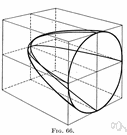# plane section

Also found in: Thesaurus, Encyclopedia.
ThesaurusAntonymsRelated WordsSynonymsLegend:
 Noun 1plane section - (geometry) the area created by a plane cutting through a solidsectionsurface area, expanse, area - the extent of a 2-dimensional surface enclosed within a boundary; "the area of a rectangle"; "it was about 500 square feet in area"geometry - the pure mathematics of points and lines and curves and surfacescross section - a section created by a plane cutting a solid perpendicular to its longest axis
Based on WordNet 3.0, Farlex clipart collection. © 2003-2012 Princeton University, Farlex Inc.
References in periodicals archive ?
For the bonded shear wall, the ductility' calculation is based on the plane section assumption and the deformation coordination assumption between reinforcement and concrete.
Denote by K([pi]) or K(U [and] V) the sectional curvature of M associated with a plane section [pi] [subset.bar] [T.sub.p]M, where {U, V} is an orthonormal basis of [pi].
Caption: Figure 5: A coronal plane section of the model coated with HA coating
If k = n then [[PI].sub.n] = [T.sub.p] (M), and if k = 2 then [[PI].sub.2] is a plane section of [T.sub.p] (M).
Based on the assumption of the plane section, the sections resistance at t moment is [4,14]
For the plane section [e.sub.i] [conjunction] [e.sub.j] of the tangent bundle TM spanned by the vectors [e.sub.i] and [e.sub.j] ([e.sub.i] [not equal to] [e.sub.j]) the scalar curvature of M is defined by [kappa] = [[summation].sup.n.sub.i,j=1] K([e.sub.i] [conjunction] [e.sub.j]), where K denotes the sectional curvature of M.
a plane section normal on the spindle axis before deformation remains plane without keeping perpendicularity
The intersection of K with a two-dimensional flat [alpha] is a plane convex body (plane section) that will be denoted by [K.sub.[alpha]].
From strains of eight discrete points, curvature, of the bridge is calculated based on plane section assumption and the curvature change at each construction stage is compared one another to verify the key feature of the proposed bridge.
If k = n then [[PI].sub.n] = [T.sub.p]M; and if k = 2 then [[PI].sub.2] is a plane section of [T.sub.p]M.
The example presented herein introduces the possibility to design the EBG only on a limited plane section, to be freely laid out where it is more appropriate without affecting other system functionalities.

Site: Follow: Share:
Open / Close